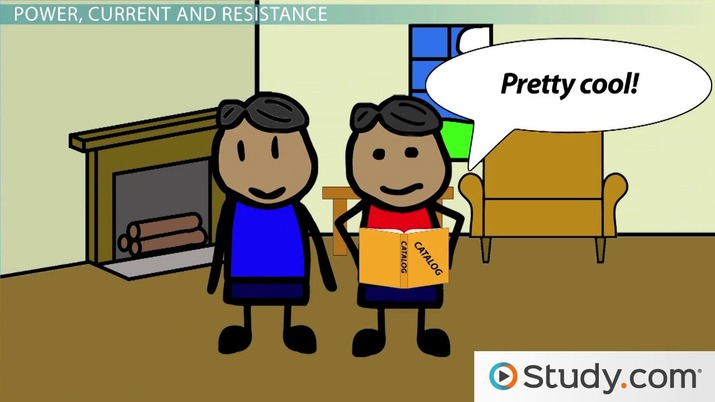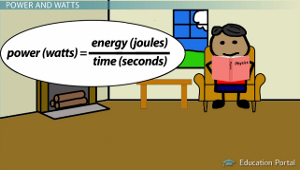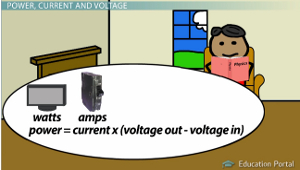# What is Electric Power?

Lesson Transcript
Instructor
Jim Heald

Jim has taught undergraduate engineering courses and has a master's degree in mechanical engineering.

Expert Contributor
Elaine Chan

Dr. Chan has taught computer and college level physics, chemistry, and math for over eight years. Dr. Chan has a Ph.D. in Chemistry from U. C. Berkeley, an M.S. Physics plus 19 graduate Applied Math credits from UW, and an A.B. with honors from U.C .Berkeley in Physics.

Power is a word we use all the time, but do you really know what it means? We'll look at how electrical power relates to voltage, current and resistance and how knowing that relationship can help you in everyday life. Updated: 10/15/2019

## A Power Problem

I recently moved into my new house, and the first thing I planned to do was to turn one of the rooms into a home theater. I'd been dreaming of this for a long time and was excited to get started. I was talking to a friend of mine who happened to be an electrician, and he warned me that I needed to be careful when choosing the equipment so that I didn't overload the circuit breakers. Heeding his warning, I went home and checked the rating of the circuit breaker for my home theater room. It said 15 amps.

Okay, I thought; now I know that together the equipment can draw no more than 15 amps of current. I got out the home theater catalog and started looking at TVs and surround sound systems. I immediately saw a problem. All of the equipment was rated in watts of power and not amps of current! I knew that power and current were related in some way, but I wasn't sure how.An error occurred trying to load this video.

Try refreshing the page, or contact customer support.

Coming up next: Magnetic Force: Definition, Poles & Dipoles

### You're on a roll. Keep up the good work!

Replay
Your next lesson will play in 10 seconds
• 0:05 A Power Problem
• 1:07 Power and Watts
• 1:50 Power, Current and Voltage
• 2:55 Power, Current and Resistance
• 5:54 Lesson Summary
Save Save

Want to watch this again later?

Timeline
Autoplay
Autoplay
Speed Speed

## Power and WattsIn search of answers, I dug out my old physics textbook and started reading. It turns out that power is a measure of how much energy is used over a period of time. There are many different units that can be used to express power, such as horsepower for cars. However, electrical power is almost universally given in watts. One watt is equal to one joule per second. This made sense when I considered that power is a measure of how much energy, measured in joules, is used per unit of time, like a second. I guess it's just easier to say watts instead of joules per second.

## Power, Current and VoltageAll this new information was great, but I still wasn't sure how to solve my problem, so I kept reading. The book went on to say that electrical power used by a device can be calculated by multiplying the current by the difference between the voltage going into and out of the device. Now I knew I was getting somewhere.

The home theater equipment was rated in watts of power and the breaker in amps of current, so all I was missing was the voltage. I knew that for devices plugged into an electrical outlet, the voltage difference through the device was equal to the voltage difference between the two slots of the outlet. Using my electrical meter, I carefully probed the electrical outlet and measured 120 volts.

With all the pieces of the puzzle, I multiplied 120 volts by the maximum current of the circuit breaker, 15 amps, and came up with 1800 watts of power. This meant that I had to select a TV and surround sound system that together used no more than 1800 watts of power.

## Power, Current and Resistance

Later that day, my electrician friend came over to see how I was coming along with picking out equipment. Excitedly, I showed him the gigantic 80-inch flat screen TV and mega-bass surround sound system I had selected from the catalog. I assured him that I had done my research and figured out that this equipment was just under the 1800-watt limit that I had calculated. 'Not so fast,' he said; 'You're forgetting something! You're not accounting for the power lost in the house wiring before it even gets to your equipment.' He was right. I hadn't even thought of this.

To unlock this lesson you must be a Study.com Member.

## Electric Power

Electric power is the time rate of change of electric energy. The units of power are watts (W), which are equal to joules per second. The power (P) is calculated by multiplying I (electric current in amperes) by V, (the potential difference in volts). When Ohms law holds, V = I * R, where R is the resistance in ohms, the power can be expressed as P = I^2 R. Power can also be expressed in terms of voltage and resistance as P = V^2 /R.

Problem 1:

Calculate the resistance of a 40-watt automobile headlight designed for 12 volts.

## Electric Energy

Electric companies charge for energy used. The total energy is the power multiplied by the time it is used. One kilowatt hour equals 1,000 watts (3,600 sec) = 3,600,000 joules.

Problem 2:

An electric heater draws 15a on a 120-volt line. How much power does it require and how much will it cost to run the heater for 30 days at three hours (3) a day, when the electric company charges \$0.105 per kilowatt hour?

Answer 1: Use the relation R = V^2 / P= 12(12) / 40 = 3.6 ohms.

Answer 2: P = IV = (15 A)120 V = 1,800 watts. The cost = (1.8)(90)(\$.105) = \$17.

### Register to view this lesson

Are you a student or a teacher?